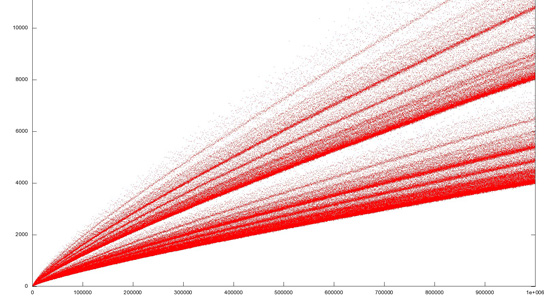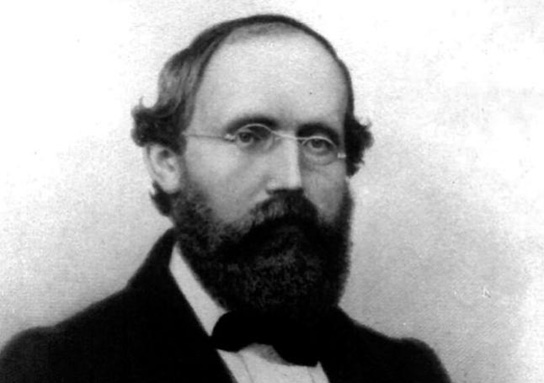# Century-Old Goldbach Weak Conjecture Closer to Being SolvedThe weak Golbach conjecture states that you can break up any odd number into the sum of, at most, three prime numbers. Prime numbers cannot be evenly divided by any other number than themselves or 1.

Fields Medalist Terence Tao of the University of California, in Los Angeles, has inched closer toward the proof. He has shown that odd numbers can be written as sums of, at most, five primes. He’s hopeful that he’ll be able to whittle this down to three. The proof has eluded numerous theorists for more than three centuries, and Tao thinks that it could be used to encrypt sensitive data more thoroughly.In the 18th-century, mathematician Christian Goldbach proposed his conjecture, which is an analog of a similar statement for even numbers, named the strong Goldbach conjecture, but which was actually formulated by his colleague Leonhard Euler. The strong version states that even numbers larger than two are the sums of two primes. The weak version would follow if the strong one were true.

The conjectures have been verified for numbers up to 19 digits. No exception has been found. The odds for larger numbers are better than smaller ones, suggesting the validity of these conjectures. A theorem from the 1930s states that there are, at most, a finite number of exceptions to the conjecture. This means that the weak Goldbach conjecture is true for sufficiently large numbers. Tao combined computer-based results that were valid for small-enough numbers to see if these would apply to large-enough numbers. He was able to improve earlier calculations by a number of tweaks, and was able to bring the two ranges to overlap, as long as 5 primes were used.

Tao thinks that he can reduce the number of primes to three in the case of the weak conjecture, but that’s not likely to help to prove the strong conjecture.

#### 2 Commentson "Century-Old Goldbach Weak Conjecture Closer to Being Solved"

1.George | November 30, 2013 at 11:47 pm | Reply

The man on the picture is Bernhard Riemann. He’s certainly not Christian Goldbach.

2.Mario Casella | September 27, 2020 at 9:24 am | Reply

Why B. Riemann picture?